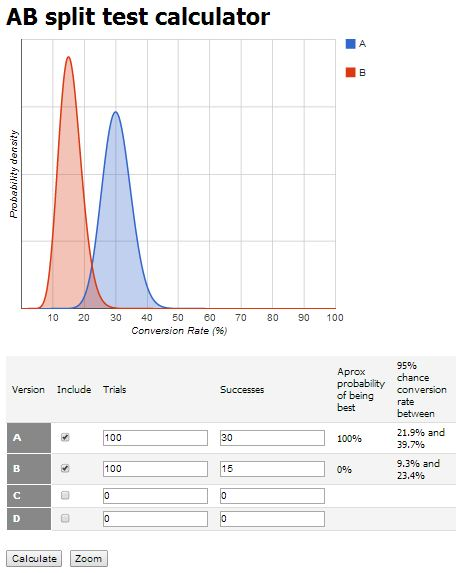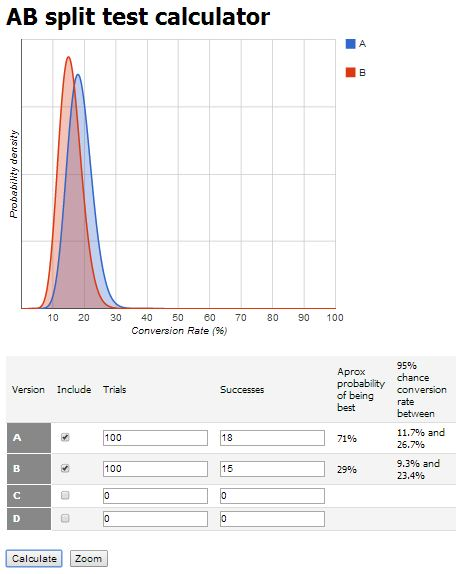﻿ An approximation to Bayesian calculator applied to AB tests probability of success - ECOMpedia

## Creeaza-ti un Cont Nou

Trebuie sa fii logat in cont sa pui o intrebare.

## Creeaza-ti un Cont Nou

Nu ai cont inca? Creează un cont nou pentru a avea acces in toate sectiunile ECOMpedia.ro.

## An approximation to Bayesian calculator applied to AB tests probability of success

I saw a recent post made by one of my friend who has a AB testing tool (aka Marketizator – a tool which does more than AB testing, you should try it) about how to compute the chances of success when you do such a test. I will make an approximation which will be very good to people doing AB Tests using tools that not compute this using a statistical approach, like Bayesian calculator. I’ll try to keep it simple; the math involved is based on a simple algebra formula.  I’ll start from this resource – AB split test graphical Bayesian calculator.fig 1

In this first image you have 2 variants A and B and both have the same chance to be the winner. We will not talk here about the conversion rate, which is 15/100 = 15% in the picture, but rather we will try to compute the probability of A having more chances to beat B variant. So, it is clear from the first picture that this chance is equally shared between A and B in the first picture. Both variants have the same chance, 50% of being the winner.fig 2

The second picture will be again very simple to understand. Now variant A has 2 times more conversions than variant B in the same conditions (the A and B were 100 times exposed to users, each one equally).  That math is very simple to understand: in case we have only 3 conversions in this experiment, A will have 2 “successes” and B will have 1 “success”. If we try to estimate a chance right now, 1 from B will go out with 1 from A, and A will have 100% chance (the 1 success that remain from the 2) to beat the B variant.fig 3

The 3rd picture will reveal the simple formula that approximate the Bayesian calculator used into the resource article (please note that the Bayesian estimation is more accurate than the formula I’ll show you). The equally chance in the case of 2 variants is 50%. If we had 3 variants the equally chance will be 33%, and so on, depends on the number of variants you use in experiment. Going back to the picture above, there is a difference of conversions which is 7 which A variant has more over the line of 50% chance (over that “15 line”, imaginary one). So the formula is 0.5 + (the difference / the smallest conversions number), which held in our case 0.5 + (7/15) = 0.96, means 96% chance to beat the version B. It is not the correct formula as you can see, Bayesian calculator is taking into account the “95% confidence level” too. But, I repeat, this is a simple approximation.fig 4

Let’s compute it again in the picture above. (18-15) / 15 = 3 / 15 = 0.2, after that 0.2 + 0.5 = 0.7, means 70% chance of beating B variant. Again, the formula you may use is 0.5 + (the diff / the small no) in percent. It can be modified easily in the case of 3/4/more variants. Another discussion is how many conversions the experiment needs (or how much time will take) in order this computation to be relevant, but that is another story, maybe another article.

I hope this article will be helpful for people using tolls that do not compute this chance, or maybe for a very short check during the experiments as you can do this math in your mind very quickly. Have fun doing tests. Always keep testing.

Si acum pentru cititorii mei vorbitori de limba Romana, cati dintre voi ar participa la un workshop / training de optimizare a conversiei de 2 zile? V-ar interesa? Unul unde sa vina mai multi vorbitori si sa va explice cum este cu AB testing-ul, cu Usability, cu mesajele trigger-uite automat de aplicatie – mailuri tranzactionale, sa va explice despre product recommendation engines?? Cati dintre voi doriti sa va cresteti conversia?